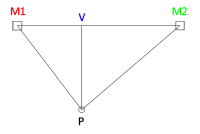The Makelangelo is a great way to start learning about robotics. It has two motors mounted at the top of a wall. The motors have bobbins wound with string. The strings are connected to a plotter, a tool that holds a pen. To draw lines the computer can control the motors, which pulls the strings and moves the pen.

## But how does that actually work?

I’ll start with the motors, then the measurements, and then the math. After that you’ll have all the theory and we can start talking about what the Arduino computer does.

### Motors

When you give power to a normal electric motor it spins as fast as it can. A lot of robotics involves stepper motors. They have a special design so that they only move one step each time they are told to, and only in the direction they are told to. I use NEMA17 stepper motors that have 400 steps per turn, or about 0.9 degrees per step. NEMA17 is a particular size. I have seen NEMA sizes from 8 all the way up to 34.

### Measurements#### Inverse Kinematics

I know how much string is reeled in or out at each step because I know the number of steps per turn and I know the circumference of the bobbin.

```STEPS = 200;
DIAMETER = 0.9;  // cm
CIRCUMFERENCE = DIAMETER * PI;
TPS = CIRCUMFERENCE / STEPS;```

I can use Pythagorus’ theorem to find the lengths of the strings because I have The distance between the motors (M1M2) and I know the position of the plotter (P) . If all of this was on a grid then I could say

```function IK(P) {
A = Px - M1x;  // same as V-M1 in the picture
B = Py - M1y;  // same as P-V in the picture
M1P = square_root(A*A+B*B);
A = Px - M2x;  // same as V-M2 in the picture
// B is the same
M2P = square_root(A*A+B*B);

return M1P,M2P;
}```

In robotics this is known as Inverse Kinematics – when you know where you want to be and you calculate how to move the motors/muscles/etc in order to get there.

#### Forward Kinematics

There’s also Forward Kinematics, when you know how far the robot moved and you need to find out where the thing has gone to. For that I use the law of cosines.

```function FK() {
A = length( P-M1 );
B = length( M1-M2 );
C = length( P-M2 );
Theta = arccos( ( A*A + B*B - C*C ) / ( 2*A*B ) );
Px = cos( Theta ) * A*A + M1;
Py = sin( Theta ) * A*A + M1;
}```

So: If you can calculate the length of string at any point then you can find the difference – we can find the moment the string length needs to change while it is moving.

#### Linear Interpolation

Let’s pretend I’m moving M (me) from point H (here) to point T (there) in one second. At any moment I can describe my position as

`M = ( T - H ) * S + H`

At the start of the second S=0 and M=T. at the middle S=0.5 and M is halfway between here and there. At S=1 M=T (I have arrived there).

### Putting it all together

If we combine this we get something like

```function DrawLineTo(T) {
start = IK( H );
tstart = millis();
loop {
S = ( millis() - tstart ) * 0.001;
now = IK( ( T - H ) * S + H );

ChangeStringLengths(now,start);
} while( S < 1 ); } function ChangeStringLengths( now, &start ) { if( now.MIP > start.MIP + TPS ) {  M1.Unwind();  start.M1P+=TPS;  }
if( now.MIP < start.MIP - TPS ) { M1.WindUp(); start.M1P-=TPS; } if( now.M2P > start.M2P + TPS ) {  M2.Unwind();  start.M2P+=TPS;  }
if( now.M2P < start.M2P - TPS ) {  M2.WindUp();  start.M2P-=TPS;  }
}
```

The rest, as they say, is details. Stepper motors can only turn so fast; the type of plotter used might change things; sometimes the now IK is more than two TPS from the current TPS; and so on.

I use a very similar technique for drawing arcs, or parts of circles. The only difference is that now I’m moving on a curve instead of a straight line.

### Arduino code

Download the arduino code here. Almost all of the code has one job: to prevent user error. The rest is comments to explain my specific implementation.

## Wait! What about the halftones?

Halftone() uses the line drawing code to fill in a square with a zigzag pattern. I tell halftone how big the square is, how thick the zigzag line is, and how much to fill in. It does the rest!

## Can you put all these parts in a kit for me?

I’ve used timing belt and pulleys instead for greater precision, and written software to make it super easy to run.

If you have any comments or questions about the robot, please feel free to post them below and I’ll be happy to answer.

## Only registered users can comment.

1.Martin Piñeyro says:

Hello,
I’m working on a plotter like this and I was wondering if I need forward kinematics at all. If my plotter takes xy coordinates as an input isn’t that enough to calculate the amount of steps each motors needed in order to get to the next point? Maybe I’m missing something important. I appreciate your comments.

2.Sky says:

Hi! Great job on the code. Unfortunately, we realize we are using a V2 driver with your V1 code. Is there a quick fix to this?

1.Dan says:

there is unofficial support for v2 in the makelangelo firmware.